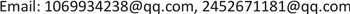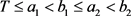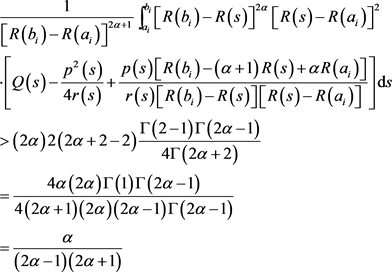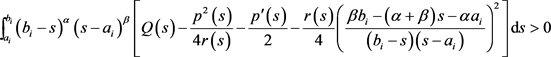﻿ 一类二阶混合非线性微分方程的振动准则 Oscillation Criteria for a Class of Se-cond-Order Mixed Nonlinear Differential Equations

Vol. 08  No. 04 ( 2019 ), Article ID: 29992 , 11 pages
10.12677/AAM.2019.84092

Oscillation Criteria for a Class of Second-Order Mixed Nonlinear Differential Equations

Jinying Lin, Tengjie Chen

School of Mathematics and Big Data, Huizhou University, Huizhou GuangdongReceived: Apr. 6th, 2019; accepted: Apr. 21st, 2019; published: Apr. 28th, 2019ABSTRACT

New oscillation criteria for a class of second-order mixed nonlinear damping equations are obtained by means of the integral averaging technique and a new kernel function combined with the Elure integral. The new results have a higher generality than some of previous results. The zero distribution information of the solution is also obtained.

Keywords:Oscillation, Second-Order, Differential Equations, Mixed Nonlinearities, Riccati Transform1. 引言

${\left(r\left(t\right){y}^{\prime }\right)}^{\prime }+p\left(t\right){y}^{\prime }+\underset{i=1}{\overset{n}{\sum }}{q}_{i}\left(t\right){|y|}^{{\alpha }_{i}-1}y=e\left(t\right)$ (1)

${\left(r\left(t\right){y}^{\prime }\right)}^{\prime }+q\left(t\right)y=e\left(t\right)$ (2)

${\left(r\left(t\right){y}^{\prime }\right)}^{\prime }+q\left(t\right)f\left(y\right)=e\left(t\right)$ (3)

1999年，Wong  和Kong  分别指出，Ei-Sayed  为方程(2)建立的区间振动准则中，由于其证明过程需要与一个常系数方程做比较而使得其结果的应用受到限制。于是，Wong  和Kong  分别建立方程(2)和方程(3)在  的情形下的区间振动准则。2003年，Yang  应用Philos  和Kong  在方程(3)中使用的技巧，给出了几个区间振动准则。其中一个Kamenevs类型的振动准则如下：

(A1) 对 $\forall T\ge {t}_{0}$$\exists T\le {a}_{1}<{b}_{1}\le {a}_{2}<{b}_{2}$ 使得

$e\left(t\right)\left\{\begin{array}{l}\le 0,t\in \left[{a}_{1},{b}_{1}\right],\\ \ge 0,t\in \left[{a}_{2},{b}_{2}\right].\end{array}$

(A2) 存在 ${c}_{i}\in \left({a}_{i},{b}_{i}\right),i=1,2$ ，使得下列不等式成立

$\frac{1}{{\left({c}_{i}-{a}_{i}\right)}^{\lambda -1}}{\int }_{{a}_{i}}^{{c}_{i}}{\left(s-{a}_{i}\right)}^{\lambda }{|e\left(s\right)|}^{1-\frac{1}{v}}{\left[Kq\left(s\right)\right]}^{\frac{1}{v}}\text{d}s\ge \frac{{\lambda }^{2}}{4\left(\lambda -1\right)}$ (4)

$\frac{1}{{\left({b}_{i}-{c}_{i}\right)}^{\lambda -1}}{\int }_{{c}_{i}}^{{b}_{i}}{\left({b}_{i}-s\right)}^{\lambda }{|e\left(s\right)|}^{1-\frac{1}{v}}{\left[Kq\left(s\right)\right]}^{\frac{1}{v}}\text{d}s\ge \frac{{\lambda }^{2}}{4\left(\lambda -1\right)}$ (5)

$\underset{t\to \infty }{\mathrm{lim}}\mathrm{sup}\frac{1}{{t}^{2\alpha +1}}{\int }_{l}^{t}{\left(t-s\right)}^{2\alpha }{\left(s-l\right)}^{2}\left[4kq\left(s\right)-{p}^{2}\left(s\right)+4\frac{t-\left(1+\alpha \right)s+\alpha l}{\left(t-s\right)\left(s-l\right)}p\left(s\right)\right]\text{d}s>\frac{4\alpha }{\left(2\alpha -1\right)\left(2\alpha +1\right)}$ (6)

(H1) 在E上 $H\left(t,t,l\right)=0$$H\left(t,l,l\right)=0$ ，且在 ${E}_{0}$$H\left(t,s,l\right)>0$

(H2) $H\left(t,s,l\right)$${E}_{0}$ 上对第二个变量存在连续的偏导函数，且存在 $h\in C\left({E}_{0},R\right)$ ，满足

$\frac{\partial H\left(t,s,l\right)}{\partial s}=H\left(t,s,l\right)h\left(t,s,l\right),\text{}\left(t,s,l\right)\in {E}_{0}$

i) $\underset{j=1}{\overset{n}{\sum }}{\alpha }_{j}{\eta }_{j}=1$

ii) $\underset{j=1}{\overset{n}{\sum }}{\eta }_{j}=1,\text{}0<{\eta }_{j}<1$

2. 主要结论

$e\left(t\right)\left\{\begin{array}{l}\le 0,t\in \left[{a}_{1},{b}_{1}\right],\\ \ge 0,t\in \left[{a}_{2},{b}_{2}\right].\end{array}$ (7)

${\int }_{{a}_{i}}^{{b}_{i}}H\left({b}_{i},s,{a}_{i}\right)\left\{Q\left(s\right)-\frac{r\left(s\right)}{4}{\left[\frac{p\left(s\right)}{r\left(s\right)}-h\left({b}_{i},s,{a}_{i}\right)\right]}^{2}\right\}\text{d}s>0$ (8)

$w\left(t\right)=\frac{r\left(t\right){y}^{\prime }\left(t\right)}{y},\text{\hspace{0.17em}}\text{\hspace{0.17em}}t\ge {T}_{1}$ (9)

${w}^{\prime }\left(t\right)=-\frac{p\left(t\right)}{r\left(t\right)}w\left(t\right)-\underset{i=1}{\overset{n}{\sum }}{q}_{i}\left(t\right){|y|}^{{\alpha }_{i}-1}-\frac{{w}^{2}\left(t\right)}{r\left(t\right)}+\frac{e\left(t\right)}{y}$ (10)

$\underset{k=1}{\overset{n}{\sum }}{C}_{k}{V}_{k}\ge \underset{k=1}{\overset{n}{\prod }}{V}_{k}^{{C}_{k}}$ (11)

$Q\left(t\right)=\underset{k=1}{\overset{n}{\prod }}{\eta }_{k}^{-{\eta }_{k}}{q}_{k}^{{\eta }_{k}}\left(t\right)$ (12)

${V}_{k}={\eta }_{k}^{-1}{q}_{k}\left(t\right){|y\left(t\right)|}^{{\alpha }_{k}-1}$ (13)

$\begin{array}{c}\underset{k=1}{\overset{n}{\sum }}{q}_{k}\left(t\right){|y\left(t\right)|}^{{\alpha }_{k}-1}=\underset{k=1}{\overset{n}{\sum }}{\eta }_{k}{\eta }_{k}^{-1}{q}_{k}\left(t\right){|y\left(t\right)|}^{{\alpha }_{k}-1}\\ \ge {\underset{k=1}{\overset{n}{\prod }}\left[{\eta }_{k}^{-1}{q}_{k}\left(t\right){|y\left(t\right)|}^{{\alpha }_{k}-1}\right]}^{{\eta }_{k}}\\ \ge \underset{k=1}{\overset{n}{\prod }}{\eta }_{k}^{-{\eta }_{k}}{q}_{k}^{{\eta }_{k}}\left(t\right){|y\left(t\right)|}^{\underset{k=1}{\overset{n}{\sum }}{\alpha }_{k}{\eta }_{k}-{\eta }_{k}}\\ =\underset{k=1}{\overset{n}{\prod }}{\eta }_{k}^{-{\eta }_{k}}{q}_{k}^{{\eta }_{k}}\left(t\right)=Q\left(t\right)\end{array}$ (14)

${w}^{\prime }\left(t\right)\le -\frac{p\left(t\right)}{r\left(t\right)}w\left(t\right)-Q\left(t\right)-\frac{{w}^{2}\left(t\right)}{r\left(t\right)}+\frac{e\left(t\right)}{y}$ (15)

${w}^{\prime }\left(t\right)\le -\frac{p\left(t\right)}{r\left(t\right)}w\left(t\right)-Q\left(t\right)-\frac{{w}^{2}\left(t\right)}{r\left(t\right)}$(16)

$\begin{array}{l}{\int }_{{a}_{1}}^{{b}_{1}}H\left({b}_{1},s,{a}_{1}\right)Q\left(s\right)\text{d}s\\ \le -{\int }_{{a}_{1}}^{{b}_{1}}H\left({b}_{1},s,{a}_{1}\right){w}^{\prime }\left(s\right)\text{d}s-{\int }_{{a}_{1}}^{{b}_{1}}\frac{p\left(s\right)H\left({b}_{1},s,{a}_{1}\right)w\left(s\right)}{r\left(s\right)}\text{d}s-{\int }_{{a}_{1}}^{{b}_{1}}\frac{H\left({b}_{1},s,{a}_{1}\right){w}^{2}\left(s\right)}{r\left(s\right)}\text{d}s\\ ={\int }_{{a}_{1}}^{{b}_{1}}H\left({b}_{1},s,{a}_{1}\right)\left\{\left[h\left({b}_{1},s,{a}_{1}\right)-\frac{p\left(s\right)}{r\left(s\right)}\right]w\left(s\right)-\frac{{w}^{2}\left(s\right)}{r\left(s\right)}\right\}\text{d}s\\ \le {\int }_{{a}_{1}}^{{b}_{1}}H\left({b}_{1},s,{a}_{1}\right)\frac{r\left(s\right)}{4}{\left[h\left({b}_{1},s,{a}_{1}\right)-\frac{p\left(s\right)}{r\left(s\right)}\right]}^{2}\text{d}s\end{array}$ (17)

${\int }_{{a}_{1}}^{{b}_{1}}H\left({b}_{i},s,{a}_{i}\right)\left\{Q\left(s\right)-\frac{r\left(s\right)}{4}{\left[h\left({b}_{i},s,{a}_{i}\right)-\frac{p\left(s\right)}{r\left(s\right)}\right]}^{2}\right\}\text{d}s\le 0$ (18)

$y\left(t\right)<0$ 时，我们可以选择 ${b}_{2}>{a}_{2}>{T}_{1}$ ，使得在区间$e\left(t\right)\ge 0$ ，那么就得到类似上述的矛盾。所以 $y\left(t\right)$ 至少有一个零解 ${t}^{*}\in \left[{a}_{2},{b}_{2}\right]$

$H\left(t,s,l\right)={\left(t-s\right)}^{\alpha }{\left(s-l\right)}^{\beta }$ ，其中 $\alpha ,\beta >1$ ，根据定理2.1，我们有以下的振动结论。

${\int }_{{a}_{i}}^{{b}_{i}}{\left({b}_{i}-s\right)}^{\alpha }{\left(s-{a}_{i}\right)}^{\beta }\left[Q\left(s\right)-\frac{r\left(s\right)}{4}{\left(\frac{p\left(s\right)}{r\left(s\right)}-\frac{\beta {b}_{i}-\left(\alpha +\beta \right)s-\alpha {a}_{i}}{\left({b}_{i}-s\right)\left(s-{a}_{i}\right)}\right)}^{2}\right]\text{d}s>0$ (19)

$H\left(t,s,l\right)={\left[R\left(t\right)-R\left(s\right)\right]}^{\alpha }{\left[R\left(s\right)-R\left(l\right)\right]}^{\beta }$ ，其中 $\alpha ,\beta >1$ ，则有

$h\left(t,s,l\right)=\frac{\beta R\left(t\right)-\left(\alpha +\beta \right)R\left(s\right)+\alpha R\left(l\right)}{r\left(s\right)\left[R\left(t\right)-R\left(s\right)\right]\left[R\left(s\right)-R\left(l\right)\right]}$

$\begin{array}{l}{\int }_{{a}_{i}}^{{b}_{i}}{\left[R\left({b}_{i}\right)-R\left(s\right)\right]}^{\alpha }{\left[R\left(s\right)-R\left({a}_{i}\right)\right]}^{\beta }\\ \cdot \left[Q\left(s\right)-\frac{r\left(s\right)}{4}{\left(\frac{p\left(s\right)}{r\left(s\right)}-\frac{\beta R\left({b}_{i}\right)-\left(\alpha +\beta \right)R\left(s\right)+\alpha R\left({a}_{i}\right)}{r\left(s\right)\left[R\left({b}_{i}\right)-R\left(s\right)\right]\left[R\left(s\right)-R\left({a}_{i}\right)\right]}\right)}^{2}\right]\text{d}s>0\end{array}$ (20)

$\begin{array}{l}\frac{1}{{\left[R\left({b}_{i}\right)-R\left({a}_{i}\right)\right]}^{\alpha +\beta -1}}{\int }_{{a}_{i}}^{{b}_{i}}{\left[R\left({b}_{i}\right)-R\left(s\right)\right]}^{\alpha }{\left[R\left(s\right)-R\left({a}_{i}\right)\right]}^{\beta }\\ \cdot \left[Q\left(s\right)-\frac{{p}^{2}\left(s\right)}{4r\left(s\right)}+\frac{p\left(s\right)\left[\beta R\left({b}_{i}\right)-\left(\alpha +\beta \right)R\left(s\right)+\alpha R\left({a}_{i}\right)\right]}{2r\left(s\right)\left[R\left({b}_{i}\right)-R\left(s\right)\right]\left[R\left(s\right)-R\left({a}_{i}\right)\right]}\right]\text{d}s\\ >\alpha \beta \left(\alpha +\beta -2\right)\frac{\Gamma \left(\beta -1\right)\Gamma \left(\alpha -1\right)}{4\Gamma \left(\alpha +\beta \right)}\end{array}$ (21)

$\begin{array}{l}{\int }_{{a}_{i}}^{{b}_{i}}{\left[R\left({b}_{i}\right)-R\left(s\right)\right]}^{\alpha -2}{\left[R\left(s\right)-R\left({a}_{i}\right)\right]}^{\beta -2}{\left[\beta R\left({b}_{i}\right)-\left(\alpha +\beta \right)R\left(s\right)+\alpha R\left({a}_{i}\right)\right]}^{2}\frac{1}{r\left(s\right)}\text{d}s\\ ={\int }_{{a}_{i}}^{{b}_{i}}{\left[R\left({b}_{i}\right)-R\left(s\right)\right]}^{\alpha -2}{\left[R\left(s\right)-R\left({a}_{i}\right)\right]}^{\beta -2}{\left\{\beta \left[R\left({b}_{i}\right)-R\left(s\right)\right]-\alpha \left[R\left(s\right)-R\left({a}_{i}\right)\right]\right\}}^{2}\text{d}R\left(s\right)\\ ={\int }_{0}^{R\left({b}_{i}\right)-R\left({a}_{i}\right)}{u}^{\beta -2}{\left[R\left({b}_{i}\right)-R\left({a}_{i}\right)-u\right]}^{\alpha -2}{\left\{\beta \left[R\left({b}_{i}\right)-R\left({a}_{i}\right)-u\right]-\alpha u\right\}}^{2}\text{d}u\\ ={\int }_{0}^{v}{u}^{\beta -2}{\left(v-u\right)}^{\alpha -2}{\left[\beta \left(v-u\right)-\alpha u\right]}^{2}\text{d}u\\ ={\beta }^{2}{\int }_{0}^{v}{u}^{\beta -2}{\left(v-u\right)}^{\alpha }\text{d}u-2\alpha \beta {\int }_{0}^{v}{u}^{\beta -1}{\left(v-u\right)}^{\alpha -1}\text{d}u+{\alpha }^{2}{\int }_{0}^{v}{u}^{\beta }{\left(v-u\right)}^{\alpha -2}\text{d}u\end{array}$ (22)

${\int }_{0}^{1}{x}^{\mu -1}{\left(1-x\right)}^{v-1}\text{d}x=\frac{\Gamma \left(\mu \right)\Gamma \left(v\right)}{\Gamma \left(\mu +v\right)}$$Re\left(\mu ,v\right)>0$

$x=\frac{u}{v}$ 我们可以得到，

${\int }_{0}^{v}{u}^{\beta -2}{\left(v-u\right)}^{\alpha }\text{d}u={v}^{\alpha +\beta -1}\frac{\Gamma \left(\beta -1\right)\Gamma \left(\alpha +1\right)}{\Gamma \left(\alpha +\beta \right)}$ (23)

${\int }_{0}^{v}{u}^{\beta -1}{\left(v-u\right)}^{\alpha -1}\text{d}u={v}^{\alpha +\beta -1}\frac{\Gamma \left(\beta \right)\Gamma \left(\alpha \right)}{\Gamma \left(\alpha +\beta \right)}$ (24)

${\int }_{0}^{v}{u}^{\beta }{\left(v-u\right)}^{\alpha -2}\text{d}u={v}^{\alpha +\beta -1}\frac{\Gamma \left(\beta +1\right)\Gamma \left(\alpha -1\right)}{\Gamma \left(\alpha +\beta \right)}$ (25)

$\begin{array}{l}{\int }_{0}^{v}{u}^{\beta -2}{\left(v-u\right)}^{\alpha -2}{\left[\beta \left(v-u\right)-\alpha u\right]}^{2}\text{d}u\\ ={\beta }^{2}{v}^{\alpha +\beta -1}\frac{\Gamma \left(\beta -1\right)\Gamma \left(\alpha +1\right)}{\Gamma \left(\alpha +\beta \right)}-2\alpha \beta {v}^{\alpha +\beta -1}\frac{\Gamma \left(\beta \right)\Gamma \left(\alpha \right)}{\Gamma \left(\alpha +\beta \right)}+{\alpha }^{2}{v}^{\alpha +\beta -1}\frac{\Gamma \left(\beta +1\right)\Gamma \left(\alpha -1\right)}{\Gamma \left(\alpha +\beta \right)}\\ =\frac{{v}^{\alpha +\beta -1}}{\Gamma \left(\alpha +\beta \right)}\left[{\beta }^{2}\Gamma \left(\beta -1\right)\Gamma \left(\alpha +1\right)-2\alpha \beta \text{ }\Gamma \left(\beta \right)\Gamma \left(\alpha \right)+{\alpha }^{2}\Gamma \left(\beta +1\right)\Gamma \left(\alpha -1\right)\right]\\ =\alpha \beta \left(\alpha +\beta -2\right)\frac{\Gamma \left(\alpha -1\right)\Gamma \left(\beta -1\right)}{\Gamma \left(\alpha +\beta \right)}{v}^{\alpha +\beta -1}\end{array}$ (26)

$\begin{array}{l}{\int }_{{a}_{i}}^{{b}_{i}}{\left[R\left({b}_{i}\right)-R\left(s\right)\right]}^{\alpha -2}{\left[R\left(s\right)-R\left({a}_{i}\right)\right]}^{\beta -2}{\left[\beta R\left({b}_{i}\right)-\left(\alpha +\beta \right)R\left(s\right)+\alpha R\left({a}_{i}\right)\right]}^{2}\frac{1}{r\left(s\right)}\text{d}s\\ =\alpha \beta \left(\alpha +\beta -2\right)\frac{\Gamma \left(\alpha -1\right)\Gamma \left(\beta -1\right)}{\Gamma \left(\alpha +\beta \right)}{\left[R\left({b}_{i}\right)-R\left({a}_{i}\right)\right]}^{\alpha +\beta -1}\end{array}$ (27)

$\begin{array}{l}\frac{1}{{\left[R\left({b}_{i}\right)-R\left({a}_{i}\right)\right]}^{\alpha +\beta -1}}{\int }_{{a}_{i}}^{{b}_{i}}{\left[R\left({b}_{i}\right)-R\left(s\right)\right]}^{\alpha }{\left[R\left(s\right)-R\left({a}_{i}\right)\right]}^{\beta }\\ \cdot \left[Q\left(s\right)-\frac{r\left(s\right)}{4}{\left(\frac{p\left(s\right)}{r\left(s\right)}-\frac{\beta R\left({b}_{i}\right)-\left(\alpha +\beta \right)R\left(s\right)+\alpha R\left({a}_{i}\right)}{r\left(s\right)\left[R\left({b}_{i}\right)-R\left(s\right)\right]\left[R\left(s\right)-R\left({a}_{i}\right)\right]}\right)}^{2}\right]\text{d}s\\ =\frac{1}{{\left[R\left({b}_{i}\right)-R\left({a}_{i}\right)\right]}^{\alpha +\beta -1}}{\int }_{{a}_{i}}^{{b}_{i}}{\left[R\left({b}_{i}\right)-R\left(s\right)\right]}^{\alpha }{\left[R\left(s\right)-R\left({a}_{i}\right)\right]}^{\beta }\\ \text{\hspace{0.17em}}\text{\hspace{0.17em}}\cdot \left[Q\left(s\right)-\frac{{p}^{2}\left(s\right)}{4r\left(s\right)}+\frac{p\left(s\right)\left[\beta R\left({b}_{i}\right)-\left(\alpha +\beta \right)R\left(s\right)+\alpha R\left({a}_{i}\right)\right]}{2r\left(s\right)\left[R\left({b}_{i}\right)-R\left(s\right)\right]\left[R\left(s\right)-R\left({a}_{i}\right)\right]}\right]\text{d}s\\ \text{\hspace{0.17em}}\text{\hspace{0.17em}}-\frac{1}{4{\left[R\left({b}_{i}\right)-R\left({a}_{i}\right)\right]}^{\alpha +\beta -1}}{\int }_{{a}_{i}}^{{b}_{i}}{\left[R\left({b}_{i}\right)-R\left(s\right)\right]}^{\alpha -2}{\left[R\left(s\right)-R\left({a}_{i}\right)\right]}^{\beta -2}\end{array}$

$\begin{array}{l}\text{\hspace{0.17em}}\text{\hspace{0.17em}}\text{ }·{\left[\beta R\left({b}_{i}\right)-\left(\alpha +\beta \right)R\left(s\right)+\alpha R\left({a}_{i}\right)\right]}^{2}\frac{1}{r\left(s\right)}\text{d}s\\ =\frac{1}{{\left[R\left({b}_{i}\right)-R\left({a}_{i}\right)\right]}^{\alpha +\beta -1}}{\int }_{{a}_{i}}^{{b}_{i}}{\left[R\left({b}_{i}\right)-R\left(s\right)\right]}^{\alpha }{\left[R\left(s\right)-R\left({a}_{i}\right)\right]}^{\beta }\\ \text{\hspace{0.17em}}\text{\hspace{0.17em}}\cdot \left[Q\left(s\right)-\frac{{p}^{2}\left(s\right)}{4r\left(s\right)}+\frac{p\left(s\right)\left[\beta R\left({b}_{i}\right)-\left(\alpha +\beta \right)R\left(s\right)+\alpha R\left({a}_{i}\right)\right]}{2r\left(s\right)\left[R\left({b}_{i}\right)-R\left(s\right)\right]\left[R\left(s\right)-R\left({a}_{i}\right)\right]}\right]\text{d}s\\ \text{\hspace{0.17em}}\text{\hspace{0.17em}}-\alpha \beta \left(\alpha +\beta -2\right)\frac{\Gamma \left(\beta -1\right)\Gamma \left(\alpha -1\right)}{4\Gamma \left(\alpha +\beta \right)}\end{array}$ (28)

$\begin{array}{l}{\int }_{{a}_{i}}^{{b}_{i}}{\left[R\left({b}_{i}\right)-R\left(s\right)\right]}^{\alpha }{\left[R\left(s\right)-R\left({a}_{i}\right)\right]}^{\beta }\\ \cdot \left[Q\left(s\right)-\frac{r\left(s\right)}{4}{\left(\frac{p\left(s\right)}{r\left(s\right)}-\frac{\beta R\left({b}_{i}\right)-\left(\alpha +\beta \right)R\left(s\right)+\alpha R\left({a}_{i}\right)}{r\left(s\right)\left[R\left({b}_{i}\right)-R\left(s\right)\right]\left[R\left(s\right)-R\left({a}_{i}\right)\right]}\right)}^{2}\right]\text{d}s>0\end{array}$

$\begin{array}{l}\frac{1}{{\left[R\left({b}_{i}\right)-R\left({a}_{i}\right)\right]}^{\text{2}\alpha +1}}{\int }_{{a}_{i}}^{{b}_{i}}{\left[R\left({b}_{i}\right)-R\left(s\right)\right]}^{\text{2}\alpha }{\left[R\left(s\right)-R\left({a}_{i}\right)\right]}^{\text{2}}\\ \cdot \left[Q\left(s\right)-\frac{{p}^{2}\left(s\right)}{4r\left(s\right)}+\frac{p\left(s\right)\left[R\left({b}_{i}\right)-\left(\alpha +1\right)R\left(s\right)+\alpha R\left({a}_{i}\right)\right]}{r\left(s\right)\left[R\left({b}_{i}\right)-R\left(s\right)\right]\left[R\left(s\right)-R\left({a}_{i}\right)\right]}\right]\text{d}s\\ >\frac{\alpha }{\left(2\alpha -1\right)\left(2\alpha +1\right)}\end{array}$ (29)(30)

$\begin{array}{l}\frac{1}{{\left[R\left({b}_{i}\right)-R\left({a}_{i}\right)\right]}^{\text{2}\beta +1}}{\int }_{{a}_{i}}^{{b}_{i}}{\left[R\left({b}_{i}\right)-R\left(s\right)\right]}^{\text{2}}{\left[R\left(s\right)-R\left({a}_{i}\right)\right]}^{\text{2}\beta }\\ \cdot \left[Q\left(s\right)-\frac{{p}^{2}\left(s\right)}{4r\left(s\right)}+\frac{p\left(s\right)\left[\beta R\left({b}_{i}\right)-\left(\beta +1\right)R\left(s\right)+R\left({a}_{i}\right)\right]}{r\left(s\right)\left[R\left({b}_{i}\right)-R\left(s\right)\right]\left[R\left(s\right)-R\left({a}_{i}\right)\right]}\right]\text{d}s\\ >\frac{\beta }{\left(2\beta -1\right)\left(2\beta +1\right)}\end{array}$ (31)

${\int }_{{a}_{i}}^{{b}_{i}}H\left({b}_{i},s,{a}_{i}\right)p\left(s\right)h\left({b}_{i},s,{a}_{i}\right)\text{d}s=-{\int }_{{a}_{i}}^{{b}_{i}}H\left({b}_{i},s,{a}_{i}\right){p}^{\prime }\left(s\right)\text{d}s$

${\int }_{{a}_{i}}^{{b}_{i}}H\left({b}_{i},s,{a}_{i}\right)\left[Q\left(s\right)-\frac{{p}^{2}\left(s\right)}{4r\left(s\right)}-\frac{{p}^{\prime }\left(s\right)}{2}-\frac{r\left(s\right){h}^{2}\left({b}_{i},s,{a}_{i}\right)}{4}\right]\text{d}s>0$ (32)(33)

$\begin{array}{l}\frac{1}{{\left[R\left({b}_{i}\right)-R\left({a}_{i}\right)\right]}^{\alpha +\beta -1}}{\int }_{{a}_{i}}^{{b}_{i}}{\left[R\left({b}_{i}\right)-R\left(s\right)\right]}^{\alpha }{\left[R\left(s\right)-R\left({a}_{i}\right)\right]}^{\beta }\\ \cdot \left[Q\left(s\right)-\frac{{p}^{2}\left(s\right)}{4r\left(s\right)}-\frac{{p}^{\prime }\left(s\right)}{2}\right]\text{d}s>\alpha \beta \left(\alpha +\beta -2\right)\frac{\Gamma \left(\beta -1\right)\Gamma \left(\alpha -1\right)}{4\Gamma \left(\alpha +\beta \right)}\end{array}$ (34)

$\begin{array}{l}\frac{1}{{\left[R\left({b}_{i}\right)-R\left({a}_{i}\right)\right]}^{\text{2}\alpha +1}}{\int }_{{a}_{i}}^{{b}_{i}}{\left[R\left({b}_{i}\right)-R\left(s\right)\right]}^{\text{2}\alpha }{\left[R\left(s\right)-R\left({a}_{i}\right)\right]}^{\text{2}}\\ \cdot \left[Q\left(s\right)-\frac{{p}^{2}\left(s\right)}{4r\left(s\right)}-\frac{{p}^{\prime }\left(s\right)}{2}\right]\text{d}s>\frac{\alpha }{\left(2\alpha -1\right)\left(2\alpha +1\right)}\end{array}$ (35)

$\begin{array}{l}\frac{1}{{\left[R\left({b}_{i}\right)-R\left({a}_{i}\right)\right]}^{2\beta +1}}{\int }_{{a}_{i}}^{{b}_{i}}{\left[R\left({b}_{i}\right)-R\left(s\right)\right]}^{2}{\left[R\left(s\right)-R\left({a}_{i}\right)\right]}^{2\beta }\\ \cdot \left[Q\left(s\right)-\frac{{p}^{2}\left(s\right)}{4r\left(s\right)}-\frac{{p}^{\prime }\left(s\right)}{2}\right]\text{d}s>\frac{\beta }{\left(2\beta -1\right)\left(2\beta +1\right)}\end{array}$ (36)

3. 例子

$\frac{\text{1}}{\mu }{y}^{″}+\frac{2}{t}{y}^{\prime }+{q}_{1}\left(t\right){|y|}^{{\alpha }_{1}-1}y+{q}_{2}\left(t\right){|y|}^{{\alpha }_{2}-1}y=\mathrm{sin}t$ (37)

${a}_{1}=2n\text{π}-\frac{\text{π}}{2},{b}_{1}={a}_{2}=2n\text{π},{b}_{\text{2}}=2n\text{π}+\frac{\text{π}}{2},{\alpha }_{1}=\frac{3}{2},{\alpha }_{2}=\frac{1}{2}$

${\eta }_{1}=\frac{1-{\alpha }_{2}}{{\alpha }_{1}-{\alpha }_{2}}=\frac{1}{2},{\eta }_{2}=\frac{{\alpha }_{1}-1}{{\alpha }_{1}-{\alpha }_{2}}=\frac{1}{2}$

$Q\left(t\right)={\eta }_{1}^{-{\eta }_{1}}{q}_{1}^{{\eta }_{1}}\left(t\right){\eta }_{2}^{-{\eta }_{2}}{q}_{2}^{{\eta }_{2}}\left(t\right)={\eta }_{1}^{-{\eta }_{1}}{\eta }_{1}{}^{{\eta }_{1}}{\mathrm{cos}}^{{\eta }_{1}}t\cdot {\eta }_{2}^{-{\eta }_{2}}{\eta }_{2}^{{\eta }_{2}}{\mathrm{cos}}^{{\eta }_{2}}t={\mathrm{cos}}^{\left({\eta }_{1}+{\eta }_{2}\right)}t=\mathrm{cos}t.$

$\begin{array}{l}{\int }_{{a}_{i}}^{{b}_{i}}{\left({b}_{i}-s\right)}^{2}{\left(s-{a}_{i}\right)}^{2}\left[Q\left(s\right)-\frac{{p}^{2}\left(s\right)}{4r\left(s\right)}-\frac{{p}^{\prime }\left(s\right)}{2}-\frac{r\left(s\right)}{4}{\left(\frac{\beta {b}_{i}-\left(\alpha +\beta \right)s-\alpha {a}_{i}}{\left({b}_{i}-s\right)\left(s-{a}_{i}\right)}\right)}^{2}\right]\text{d}s\\ ={\int }_{2n\text{π}-\frac{\text{π}}{2}}^{2n\text{π}}{\left(2n\text{π}-s\right)}^{2}{\left(s-2n\text{π}+\frac{\text{π}}{2}\right)}^{2}\left[Q\left(s\right)-\frac{{p}^{2}\left(s\right)}{4r\left(s\right)}-\frac{{p}^{\prime }\left(s\right)}{2}-r\left(s\right){\left(\frac{2n\text{π}-2s-2n\text{π}+\frac{\text{π}}{2}}{\left(2n\text{π}-s\right)\left(s-2n\text{π}+\frac{\text{π}}{2}\right)}\right)}^{2}\right]\text{d}s\\ ={\int }_{0}^{\frac{\text{π}}{2}}{\left(\frac{\text{π}}{2}-s\right)}^{2}{s}^{2}\mathrm{sin}s\text{d}s+{\int }_{0}^{\frac{\text{π}}{2}}{\left(\frac{\text{π}}{2}-s\right)}^{2}\left(1-\mu \right)\text{d}s-{\int }_{0}^{\frac{\text{π}}{2}}\frac{1}{\mu }{\left(\frac{\text{π}}{2}-2s\right)}^{2}\text{d}s\\ =\left(-\frac{{\text{π}}^{2}}{2}-6\text{π}+24\right)-\frac{{\text{π}}^{3}}{24}\left(1-\mu \right)-\frac{1}{\mu }\cdot \frac{{\text{π}}^{3}}{24}\\ =-\frac{{\text{π}}^{2}}{2}-6\text{π}+24-\frac{{\text{π}}^{3}}{24}+\frac{{\text{π}}^{3}}{24}\mu -\frac{1}{\mu }\cdot \frac{{\text{π}}^{3}}{24}>0\end{array}$

Oscillation Criteria for a Class of Se-cond-Order Mixed Nonlinear Differential Equations[J]. 应用数学进展, 2019, 08(04): 815-825. https://doi.org/10.12677/AAM.2019.84092

1. 1. Kartsatos, A.G. (1972) Maintenance of Oscillations under the Effect of a Periodic Forcing Term. Proceedings of the American Mathematical Society, 33, 377-383. https://doi.org/10.1090/S0002-9939-1972-0330622-0

2. 2. Wong, J.S.W. (1988) Second Order Nonlinear Forced Oscillations. SIAM Journal on Mathematical Analysis, 19, 667-675. https://doi.org/10.1137/0519047

3. 3. Elbert, A. (1998) Oscillation/Nonoscillation for Linear Second Order Dif-ferential Equation. Journal of Mathematical Analysis and Applications, 226, 207-219. https://doi.org/10.1006/jmaa.1998.6059

4. 4. Ei-Sayed, M.A. (1993) An Oscillation Criteria for a Forced Se-cond-Order Linear Differential Equation. Proceedings of the American Mathematical Society, 118, 813-817. https://doi.org/10.2307/2160125

5. 5. Nasr, A.H. (1998) Sufficient Conditions for the Oscillation of Forced Su-per-Linear Second Order Differential Equation with Oscillatory Potential. Proceedings of the American Mathematical Society, 126, 123-125. https://doi.org/10.1090/S0002-9939-98-04354-8

6. 6. Wong, J.S.W. (1999) Oscillation Criteria for a Forced Se-cond Order Linear Differential Equation. Journal of Mathematical Analysis and Applications, 231, 235-340. https://doi.org/10.1006/jmaa.1998.6259

7. 7. Kong, Q. (1999) Interval Criteria for Oscillation of Second Order Linear Differential Equations. Journal of Mathematical Analysis and Applications, 229, 258-270. https://doi.org/10.1006/jmaa.1998.6159

8. 8. Yang, Q. (2003) Interval Oscillation Criteria for a Forced Second Order Nonlinear Ordinary Differential Equations with Oscillatory Potential. Applied Mathematics and Computation, 135, 49-64. https://doi.org/10.1016/S0096-3003(01)00307-1

9. 9. Philos, Ch.G. (1989) Oscillation Theorems for Linear Dif-ferential Equations of Second Order. Archiv der Mathematik (Basel), 53, 483-492. https://doi.org/10.1007/BF01324723

10. 10. Sun, Y.G. (2004) New Kamenev-Type Oscillation Criteria for Se-cond-Order Nonlinear Differential Equations with Damping. Journal of Mathematical Analysis and Applications, 291, 341-351. https://doi.org/10.1016/j.jmaa.2003.11.008

11. 11. Dube, S.G.A. and Mingarelli, A.B. (2005) Nonlinear Functions and a Theorem of Sun. Journal of Mathematical Analysis and Applications, 308, 208-220. https://doi.org/10.1016/j.jmaa.2004.11.060

12. 12. Zhuang, R.K. and Wu, H.W. (2011) New Oscillation Criteria Related to Euler’s Integral for Certain Nonlinear Differential Equation. Annals of Differential Equations, 27, 111-118.

13. 13. Yang, Q.G. (2004) Oscillation of Self-Adjoint Matrix Hamiltonian Systems. Journal of Mathematical Analysis and Applications, 296, 110-130. https://doi.org/10.1016/j.jmaa.2004.03.052

14. 14. Yang, Q.G. (2007) On the Oscillation of Certain Nonlinear Neutral Partial Differential Equations. Applied Mathematics Letters, 20, 900-907. https://doi.org/10.1016/j.aml.2006.11.001